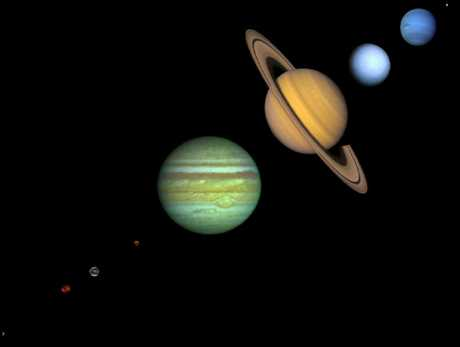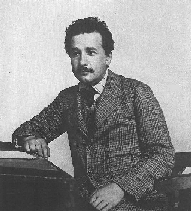Albert Einstein and
the Theory of RelativityAlbert Einstein1879-1955
Newton's theory of gravitation was soon accepted without question, and it remained unquestioned until the beginning of this century. Then Albert Einstein shook the foundations of physics with the introduction of his Special Theory of Relativity in 1905, and his General Theory of Relativity in 1915 The first showed that Newton's Three Laws of Motion were only approximately correct, breaking down when velocities approached that of light. The second showed that Newton's Law of Gravitation was also only approximately correct, breaking down in the presence of very strong gravitational fields.

## Special Relativity

As a patent clerk in Switzerland, Einstein began to think about how moving observers see events differently from stationary observers. He was led to the

FIRST POSTULATE OF SPECIAL RELATIVITY: observers can never detect uniform motion except relative to other objects.

This is part of our common experience. When you sit in a train waiting for it to go, and the train on the adjacent track starts to move, there are sometimes a few moments when you are not sure which train is moving. It is only after you see your absence of motion with respect to background objects that you realize the other train is moving.

But if you are at rest or your are moving at a constant velocity in deep space and you see another space ship pass you by moving at a constant velocity, you would not be able to tell which spaceship is really moving. This means there is NO SUCH THING AS ABSOLUTE REST, "everything is relative." Another way to say this is that the laws of physics do not distinguish between observers moving at a CONSTANT VELOCITY with respect to each other.

SECOND POSTULATE OF SPECIAL RELATIVITY: Unlike the velocity of massive objects, the speed of light is a constant and is the same for all observers independent of their CONSTANT VELOCITY toward or away from the light source.

Believe it or not, all of the above was enough for Einstein to come up with his famous equation E=mc2. (I leave it as a homework problem for you to do the same---just kidding!)

Part of the reason for this result is that if a massive object is moving from the point of view of one observer, but at rest as seen by another observer, then one observer would seem to measure zero energy of the object while the other observer would measure a finite energy. It turns out that for the laws of physics to be consistent in the two "reference frames" of two observers moving with constant speed with respect to each other the has to be an energy associated with a body at rest, not just a body in motion.

All of these effects are only when the velocity of objects approach the speed of light. The effects are hard to understand and feel in our daily lives because we are always experiencing much smaller velocities at which Newtonian physics dominates.

## General Relativity

The General Theory of Relativity is even more subtle and even farther beyond the scope of this course. Nevertheless, some of the basic ideas can be described.

First note that SPECIAL RELATIVITY effects show up for fast moving objects which are in relative motion but where the relative motion has CONSTANT VELOCITY. GENERAL RELATIVITY INCORPORATES FAST MOTIONS AND ACCELERATION.

# General Relativity: the Principle of Equivalence

Einstein first noted that freely falling in a gravitational field results in a constant acceleration (velocity changes but at a constant rate). He then realized that it is impossible for an observer to distinguish between freely falling in a gravitational field, and some other mechanism of uniform acceleration such as a rocket. This is the PRINCIPLE OF EQUIVALENCE

Einstein was then led to consider that since acceleration describes how objects move through space and time, and free fall in gravity and any uniform acceleration were indistinguishable, that gravity's effect on objects may actually be describable by it direct influence on space itself. This turned out to be a profound insight.

A physical picture of what is going on is something like the following: Consider a very large trampoline with nothing on the trampoline pad. The trampoline pad remains flat and parallel to the ground. Now place a heavy bowling ball at the center of the trampoline pad. The center of the pad will sag downward. If we assume the analogy that the trampoline pad represents space-time, and the bowling ball a gravitating object, then the sagging of the trampoline represents the curvature of space time under the influence of gravity. We can now see that if we take a lighter ball, and place it at the edge of the trampoline bad, it will roll down toward the bowling ball. This attraction to the bowling ball is because the path toward the bowling ball through space is favorably curved. In general relativity, however, it is not only balls that would follow that curved path but light as well.

### Consequences of the Principle of Equivalence

Said another way, as light passes a massive object, the path of light is actually bent by a gravitational field. This effect is even measurable during a solar eclipse. Stars whose locations we know to be behind the position of the sun are actually observable during a solar eclipse because the light is bent around on a curved path!

Here, I summarize the differences between Newton's theory of gravitation and the theory of gravitation implied by the General Theory of Relativity. They make essentially identical predictions as long as the strength of the gravitational field is weak, which is our usual experience. However, there are crucial predictions where the two theories diverge, and thus can be tested with careful experiments.1. The orientation of Mercury's orbit is found to precess in space over time, as indicated in the adjacent figure (the magnitude of the effect is greatly exaggerated in this figure). This is commonly called the "precession of the perihelion", because it causes the position of the perihelion to move. Only part of this can be accounted for by perturbations in Newton's theory. There is an extra 43 seconds of arc per century in this precession that is predicted by the Theory of General Relativity and observed to occur (a second of arc is 1/3600 of an angular degree). This effect is extremely small, but the measurements are very precise and can detect such small effects very well.

2. Einstein's theory predicts that the direction of light propagation should be changed in a gravitational field, contrary to the Newtonian predictions. Precise observations indicate that Einstein is right, both about the effect and its magnitude. A striking consequence is gravitational lensing.

3. The General Theory of Relativity predicts that light coming from a strong gravitational field should have its wavelength shifted to larger values (what astronomers call a "red shift"), again contrary to Newton's theory. Once again, detailed observations indicate such a red shift, and that its magnitude is correctly given by Einstein's theory.

## The Modern Theory of Gravitation

And there is stands to the present day. Our best current theory of gravitation is the General Theory of Relativity. However, only if velocities are comparable to that of light, or gravitational fields are much larger than those encountered on the Earth, do the Relativity theory and Newton's theories differ in their predictions. Under most conditions Newton's three laws and his theory of gravitation are adequate. We shall return to this issue in our subsequent discussion of cosmology.

For interested students, more about Einstein and his work see Albert Einstein Online